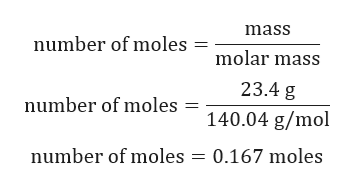# Calculate the molarity of a solution made by dissolving 23.4 g of sodium sulphate in enough water to form 125 mL of solution.

Question
1 views
• Calculate the molarity of a solution made by dissolving 23.4 g of sodium sulphate in enough water to form 125 mL of solution.
check_circle

Step 1

Given:

Mass of sodium sulphate = 23.4 g.

Volume of sodium sulphate solution = 125 mL = 0.125 L

Step 2

Calculation for numb...help_outlineImage Transcriptionclosemass number of moles molar mass 23.4 g number of moles 140.04 g/mol number of moles = 0.167 moles fullscreen

### Want to see the full answer?

See Solution

#### Want to see this answer and more?

Solutions are written by subject experts who are available 24/7. Questions are typically answered within 1 hour.*

See Solution
*Response times may vary by subject and question.
Tagged in

### Chemistry